# Constructing Circles

Constructing Circles

Circles are everywhere around us. A ball, a pizza, a pie, a wheel, a plate, a coin, and so on are a few circular objects that we come across in our daily life. We can construct a circle by tying 2 pencils at their ends together using a string. Have one pencil fixed at the center point and then run the second pencil along. We obtain a circle. Here is the simulation that shows how interestingly, a circle is been constructed.

What do you observe? The distance from the center point to any point on the circle is always the same. In this mini-lesson, let's learn how to construct circles. In the process of learning the construction, let us also learn about circles, points, collinear points, non-collinear points, perpendicular bisector, equidistant, the center of the circle, and the radius of the circle.

## Lesson Plan

 1 How Do You Construct a Circle? 2 Thinking Out of The Box! 3 Important Notes on Constructing Circles 4 Solved Examples on Constructing Circles 5 Interactive Questions on Constructing Circles

## How Do You Construct a Circle?

Before constructing a circle, we need to know about the components of a circle.

### Circle and its Parts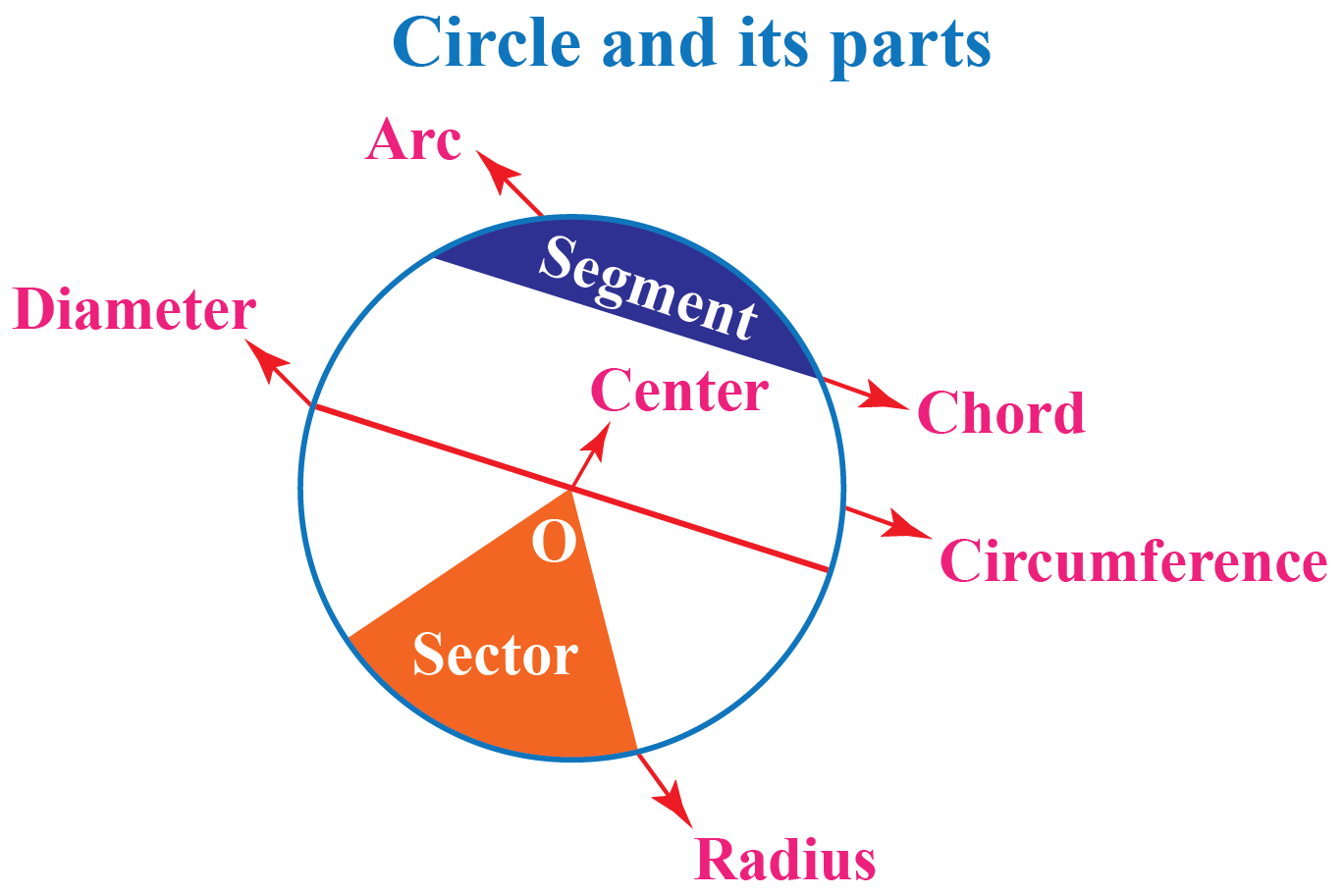Parts of the circle Explanation
Center The distance from the center of the circle to any point on the circle is the same throughout.
Circumference The distance along the circle is called its circumference
Radius The distance from the center to any point on the circle is the radius of the circle.
Diameter Is the biggest chord and is twice the radius.
Chord A chord is a line segment that connects any two points on the circumference of the circle.
Arc A part of the circumference is called an arc.
Segment The region subtended between an arc and a chord is the segment.
Sector The region subtended between two radii and an arc is the sector.

### Construction of a Circle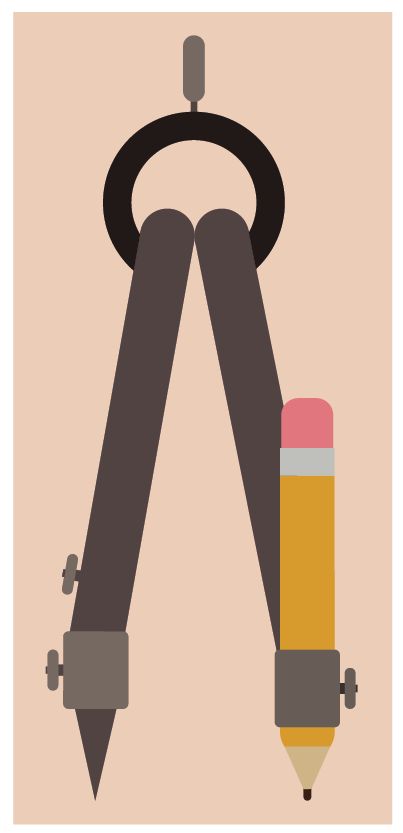• Mark a center point.
• Measure the distance between the tips of the compass and the pencil using the ruler to align with the desired radius.
• Place the metal tip on the center point.
• Keeping the metal tip constant at the center point, rotate the pencil.
• You get a circle!

Thus a circle is constructed.

## How to Construct a Circle Using a Compass?

Through a point in the plane, infinitely many lines can pass. However, through two distinct points in the plane, exactly one line can pass. That is two distinct points uniquely determine a line.

What happens in the case of circles? How many points are at least required to uniquely determine a circle?

It should be obvious that through one point, infinitely many circles can pass.

Even through two points, infinitely many circles can pass.

Let us now see how we can actually construct a unique circle passing through three distinct non-collinear points. The following figure shows three such points, A, B, and C: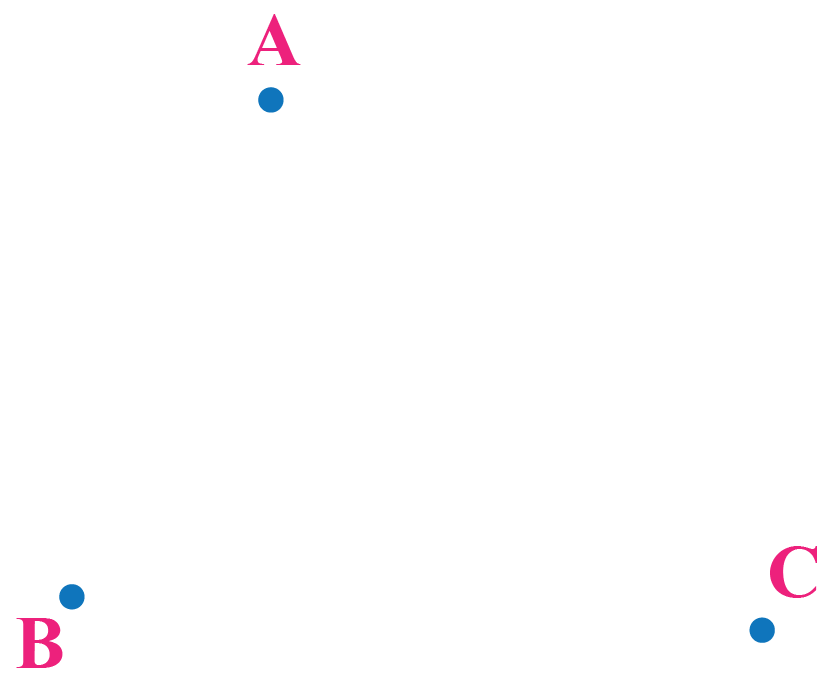The center of that circle must be equidistant from all three points.

This means that we have to locate that point in the plane (call it O) such that OA = OB = OC.

To locate O, recall that any point which is equidistant from two fixed points must lie on the perpendicular bisector of the segment joining those points. Therefore, we proceed as follows:

1. Join the points A and B and draw a perpendicular bisector $$P_1$$ of AB.

Any point on $$P_1$$ will be equidistant from A and B: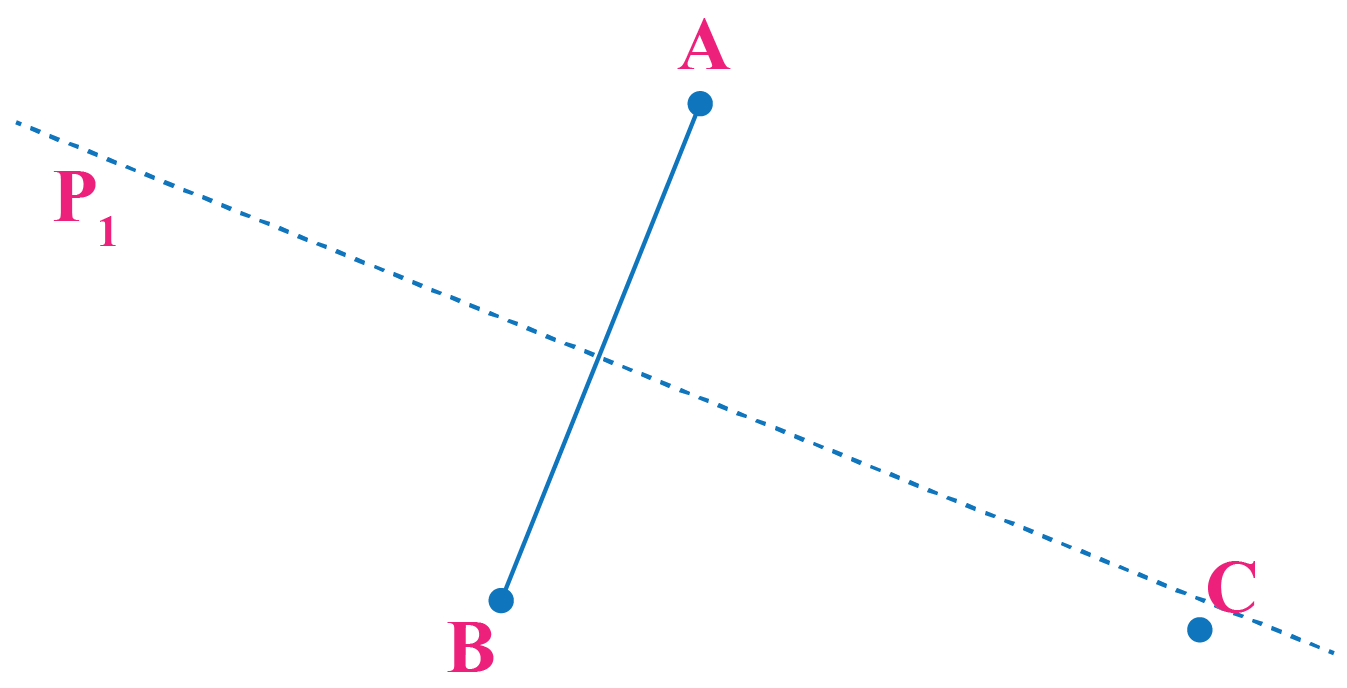2. Join B and C and draw a perpendicular bisector $$P_2$$ of BC.

Any point on $$P_2$$ will be equidistant from B and C: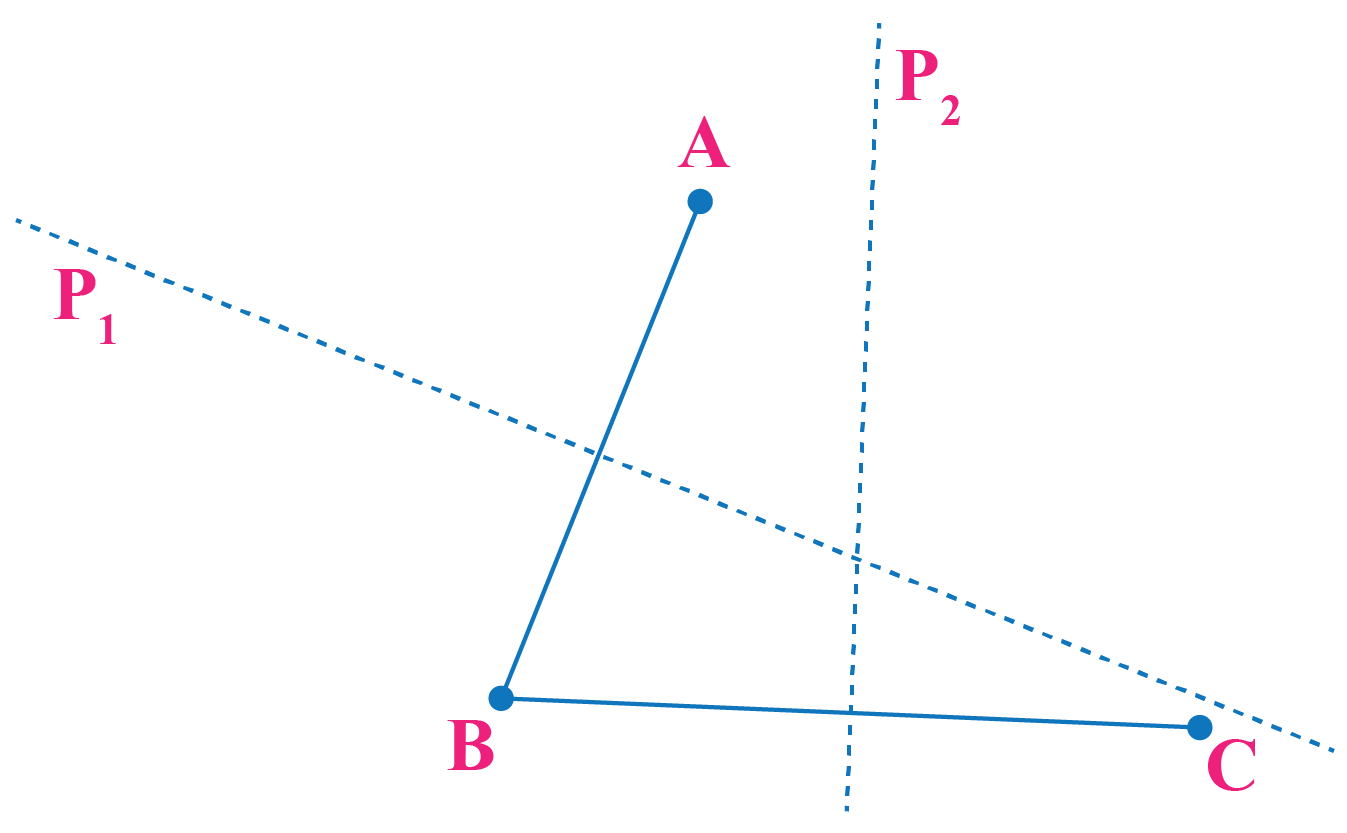3. Mark the point of intersection of $$P_1$$ and $$P_2$$ as O.

This point O is equidistant from all of A, B, and C.

Let this be the center of the circle.

4. We draw a circle with O as the center, using the compass with the radius as the measure of length OA (or OB or OC).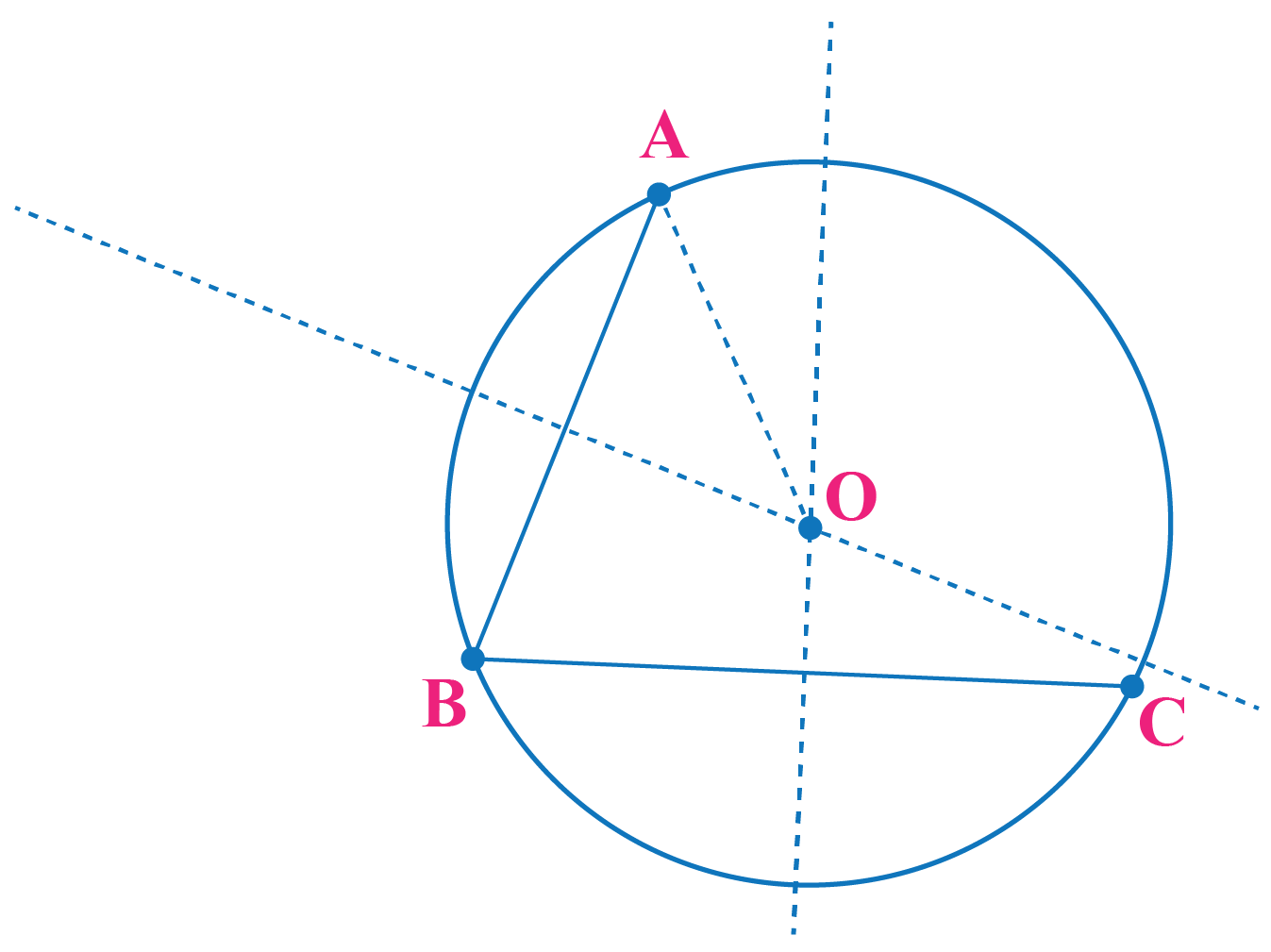Think Tank

1. Can two circles intersect in more than 2 points?

2. Why would two circles that have a common arc, must coincide?

3. How many points can be equidistant from 3 non-collinear points?

### Thus we state the theorem as:

Given any three non-collinear points A, B, and C, there exists a unique circle passing through them.

Find in the simulation how the circle is constructed using 3 distinct non-collinear points.

Circles
grade 9 | Questions Set 2
Circles
grade 9 | Questions Set 1
Circles
Circles
More Important Topics
Numbers
Algebra
Geometry
Measurement
Money
Data
Trigonometry
Calculus
More Important Topics
Numbers
Algebra
Geometry
Measurement
Money
Data
Trigonometry
Calculus